## 1. Introduction

In this tutorial, we’ll explore the Random Sample Consensus (RANSAC) algorithm. It describes a method to detect outliers in a dataset using an iterative approach.

## 2. Motivation

Outliers appear in datasets for various reasons, including measurement errors and wrong data entry. They may also be inherent to the data at hand.

### 2.1. An Example

In the data shown we see a typical dataset encountered i.e. in signal processing: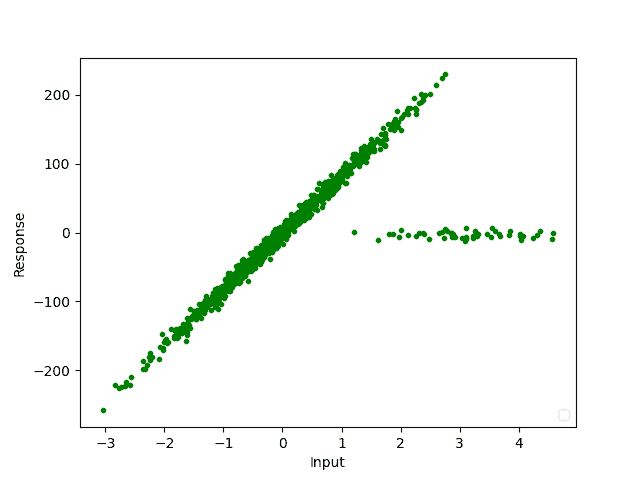The outliers are easy to spot visually, we mark them in purple: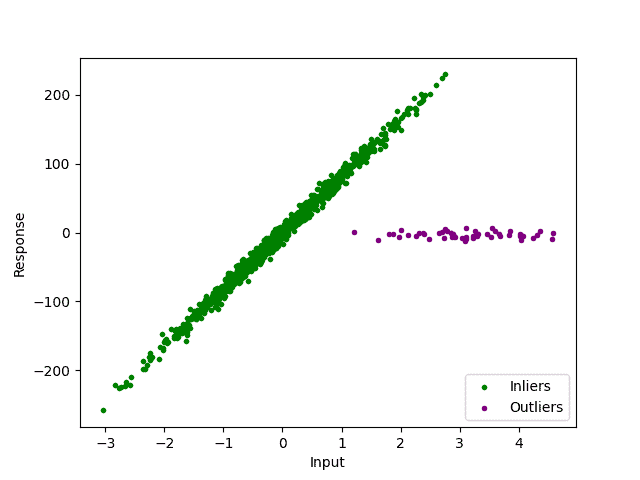In this example, it’s easy to see the outliers. In other cases, we might not even be able to visualize them in two dimensions. Furthermore, we certainly want an algorithm that detects our outliers rigorously and automatically.

### 2.2. Further Processing

We can also see that the outliers prevent us from further data processing. As an example, we have a look at a linear regression: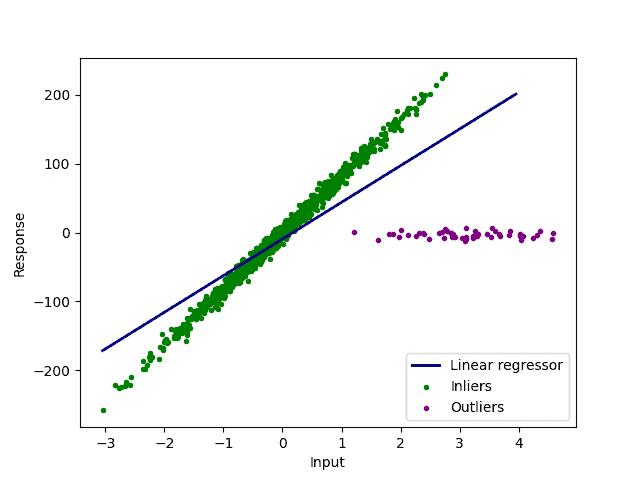As we can see, the linear regression fails to describe our data correctly.

What we do want in this example is a linear regression, that only goes through our inliers: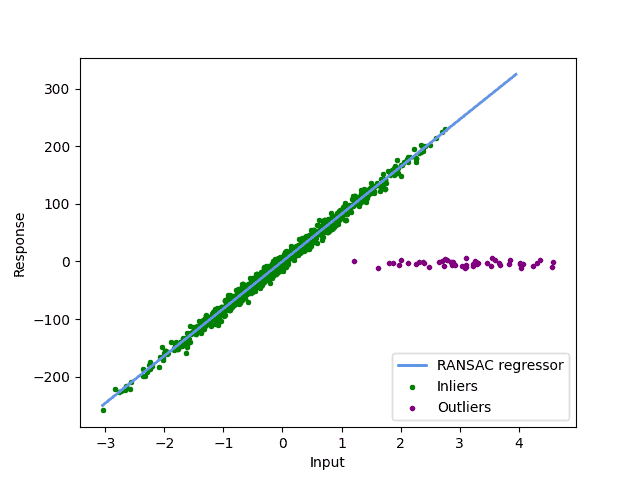## 3. Eliminating Outliers With the RANSAC Algorithm

To detect our outliers correctly and to build a model that ignores them in computation, we use the RANSAC algorithm. It works by taking a random subset of our given data and creating a model from it. Then we check how well the whole dataset fits the model. We repeat these steps until we have found a model that fits our data well. In this process, we mark all points that are not close to it as outliers.

### 3.1. The Implementation

Let’s take a closer look at the algorithm:In the center of the algorithm is our “for” loop. In this loop, we select a random subset of our data, having the previously chosen size. For this data, we fit our model. This can be any kind of model, for example, a simple linear regression.

After fitting the model, we check how far away all the other points from our original dataset are with respect to the model in our second for loop. For this distance, we use the parameteras a threshold. From these points, we create a new List, called tempInlierAdd.

If this list has a size larger than our parameter, we can consider it as a candidate for an appropriate model. Then we create a model from tempInlier and tempInlierAdd and compare it to the best model from our previous iterations.

### 3.2. Model Parameters – Threshold

To determine how far how away from our fitted line our points can be to still consider them as inliers, we use the parameteras a threshold: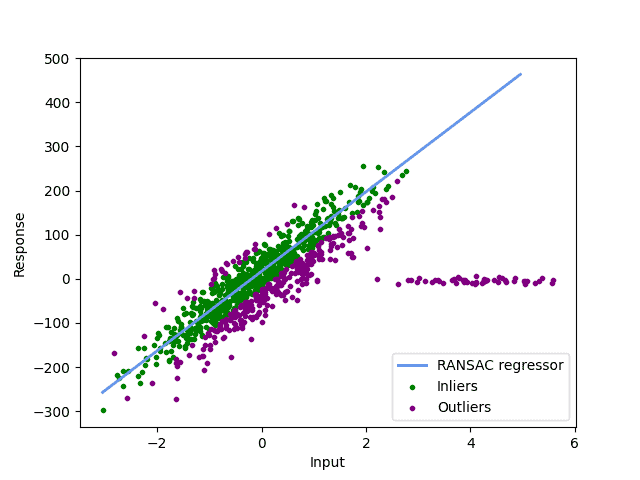If our threshold is chosen too small, as in our picture, we may detect too many points as inliers. In most RANSAC implementations, the threshold is calculated as the median of y-values from our dataset. If no threshold is given by the user. Changing the threshold manually in our example yields a much more reasonable outlier classification: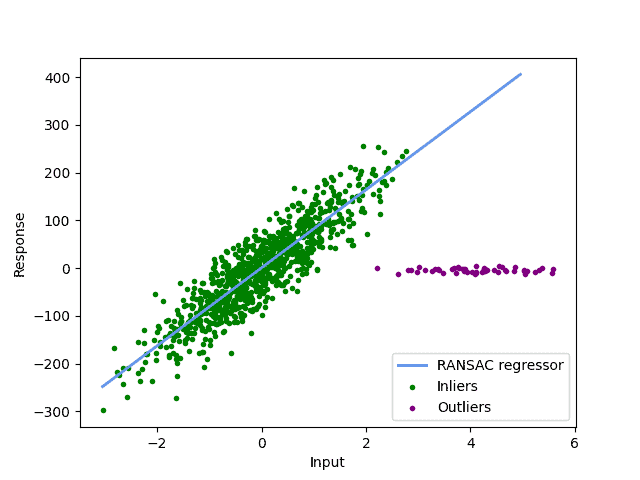A wrong threshold can not only detect the wrong outliers, but it can also result in a wrong data model. Thus, it is essential to choose it correctly.

### 3.3. Model Parameters – Number of Iterations

The higher the number of iterations, the higher the probability that we detect a subset without any outliers in it. We can use a result from statistics, that uses the ratio of inliers to total points, the number of data pointswe need for our model calculation and the probabilitythat we encounter a subset without outliers in it:As we can see with a simple example of,and, we need roughly 41 iterations to obtain a model without outliers with a probability of 99%.